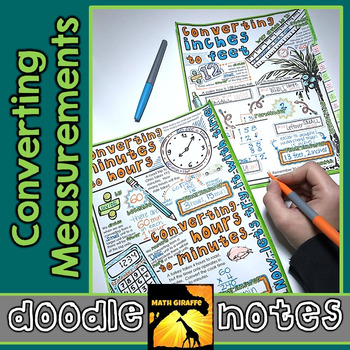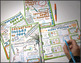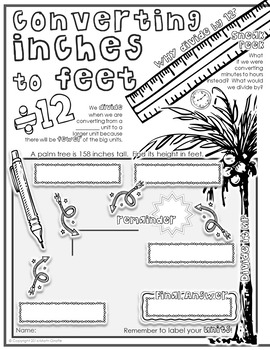# Converting Measurements Doodle NotesSubject
Resource Type
File Type

PDF

(5 MB|4 plus answer keys & info)
Standards
• Product Description
• StandardsNEW
Measurement Conversions - Multiplying & Dividing to go between Feet and Inches, and Minutes, Seconds, and Hours "doodle notes" -

When students color or doodle in math class, it activates both hemispheres of the brain at the same time. There are proven benefits of this cross-lateral brain activity:
- new learning
- relaxation (less math anxiety)
- visual connections
- better memory & retention of the content!

Students fill in the sheets, answer the questions, and color, doodle or embellish. Then, they can use it as a study guide later on.

Content includes:
- Converting between units of length - feet and inches
- How, when, and why to multiply versus divide by 12 for lengths
- Using remainders for leftover small units
- Converting between units of time - minutes, seconds, and hours
- How, when, and why to multiply or divide once or twice by 60 for different time units and going in different directions for conversions
- Sums and differences with units of length (ex: 1 foot, 3 inches + 3 feet, 11 inches)
- Visual triggers and models for concept & retention
- generic labeling too to remember the general format for small units versus large units
- Practice & Examples
- Word problems with unit conversions
- Mixed practice page

You might also like:

Christmas EquationsPrime Factorization GridWords

Coordinate PLANES: Paper Airplanes from Plotting Points

Use the four operations to solve word problems involving distances, intervals of time, liquid volumes, masses of objects, and money, including problems involving simple fractions or decimals, and problems that require expressing measurements given in a larger unit in terms of a smaller unit. Represent measurement quantities using diagrams such as number line diagrams that feature a measurement scale.
Know relative sizes of measurement units within one system of units including km, m, cm; kg, g; lb, oz.; l, ml; hr, min, sec. Within a single system of measurement, express measurements in a larger unit in terms of a smaller unit. Record measurement equivalents in a two-column table. For example, know that 1 ft is 12 times as long as 1 in. Express the length of a 4 ft snake as 48 in. Generate a conversion table for feet and inches listing the number pairs (1, 12), (2, 24), (3, 36),...
Total Pages
4 plus answer keys & info
Included
Teaching Duration
45 minutes
Report this Resource to TpT
Reported resources will be reviewed by our team. Report this resource to let us know if this resource violates TpT’s content guidelines.